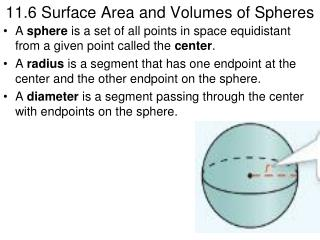DownloadDownload Presentation11.6 Surface Area and Volumes of Spheres

# 11.6 Surface Area and Volumes of Spheres

Télécharger la présentation## 11.6 Surface Area and Volumes of Spheres

- - - - - - - - - - - - - - - - - - - - - - - - - - - E N D - - - - - - - - - - - - - - - - - - - - - - - - - - -
##### Presentation Transcript

1. 11.6 Surface Area and Volumes of Spheres • A sphere is a set of all points in space equidistant from a given point called the center. • A radius is a segment that has one endpoint at the center and the other endpoint on the sphere. • A diameter is a segment passing through the center with endpoints on the sphere.

2. 11.6 Surface Area and Volumes of Spheres • When a plane and a sphere intersect in more than one point, the intersection is a circle. If the center of the circle is also the center of the sphere, it is called a great circle. • The circumference of a great circle is the circumference of the sphere. • A great circle divides a sphere into two hemispheres.

3. Theorem 11.10 Surface Area of a Sphere • The surface area of a sphere is four times the product of pi and the square of the radius of the sphere.

4. Finding the Surface Area of a Sphere • What is the surface area of the sphere in terms of pi?

5. Theorem 11.11 Volume of a Sphere • The volume of a sphere is four thirds the product of pi and the cube of the radius of the sphere.

6. Finding the Volume of a Sphere • What is the volume of the sphere in terms of pi?

7. More Practice!!!!! • Homework – Textbook p. 737 #6 – 22 even.